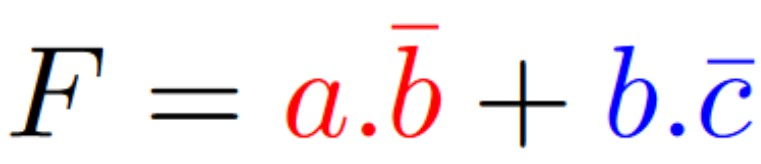# Principles of Combinatorial Logic

Theory
Combinatorial logic is used as a basis for the design of functional programming languages. It responds to a theoretical model. The information is of binary type. It is represented by electrical signals with 2 states {0; 1}.

A logical function defines the state of output variables according to the combination of input variables, without taking into account the time. The output states depend directly to the input variables. For different variables:

• Input variables: signals from other systems or control elements, such as push buttons, switches, sensors, identified or known a priori.

• Output variables: signals to activate or not the control of systems, such as lights, cylinders, motors.

More generally, combinatorial logic allows the realisation of logic functions such as ALU (Arithmetic Logic Unit), transcoders, 7 segment displays, etc.

There are 3 basic logic functions to know which allow to express all logic functions. In order to express their functionality, we use truth tables. A truth table is a mathematical table that allows us to semantically represent logical expressions and to specify their value for each combination of inputs.

Elementary functions

•  Logic function NOT

Considering the binary variable x. The output S of the logic function NOT is specified in the truth table: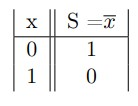• Logic function AND
Considering two binary variables x and y. The output S of the logic function AND is specified in the truth table: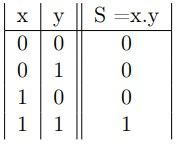`.’ is the operator AND which is different to the mathematical operator `.’, i.e. multiplication in arithmetic.

• Logic function OR
Considering two binary variables x and y. The output S of the logic function OR is specified in the truth table: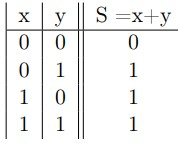`+’ s the operator OR which is different to the mathematical operator `+’, i.e. addition in arithmetic.

Other logic functions

• The logic function NAND (NOT AND)
Considering two binary variables x and y. The output S of the logic function NAND is specified in the truth table: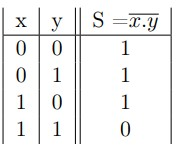• The logic function NOR (NOT OR)
Considering two binary variables x and y. The output S of the logic function NOR is specified in the truth table: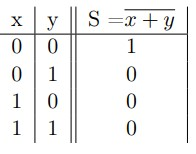• The logic function Exclusive OR
Considering two binary variables x and y. The output S of the logic function is specified in the truth table: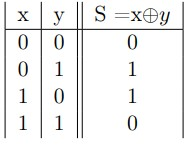• The logic function Exclusive AND
Considering two binary variables x and y. The output S of the logic function is specified in the truth table: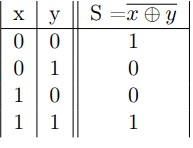Logic functions

The logic functions to be coded are often more complex. They can integrate more than 2 input variables. However, the approach is the same. It is necessary to determine their truth table, then to simplify the expressions obtained with the help of Boolean algebra or Karnaugh table.

An example of a logic function is given: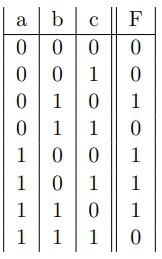The function F is determined according to combinations of inputs leading to its activation. Hence: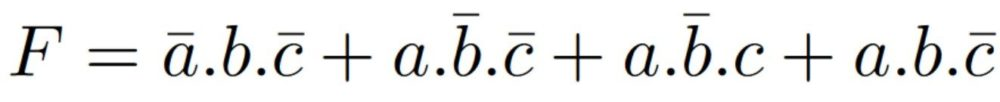This function can be code directly. However, a simplified version is preferred helping the coding and limiting errors. The graphical tool of Karnaugh is very helpful.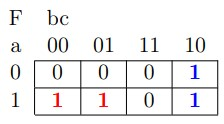In this case, two groups are possible, one with the `1′ in red, second with `1′ in blue, allowing the simplification of the function: